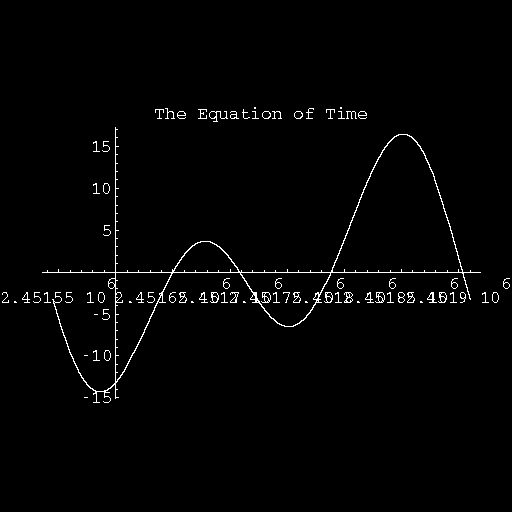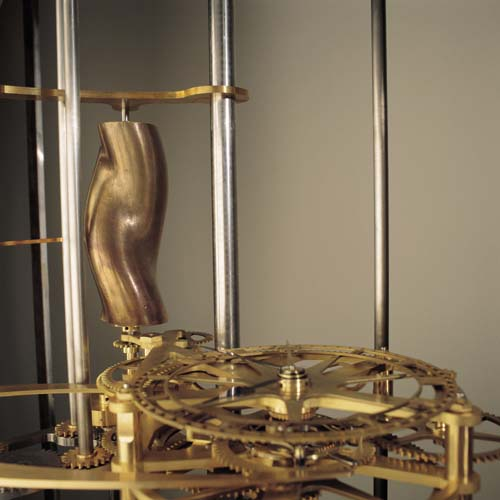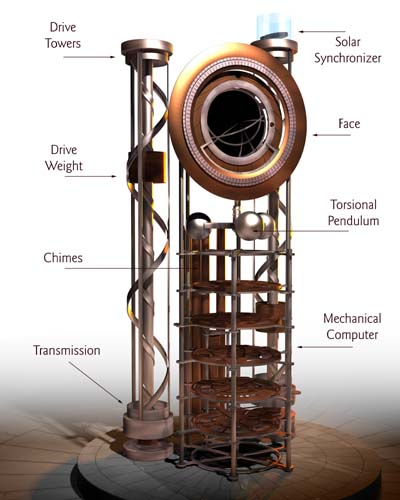#A Cam for the Clock

The Clock of the Long Now is a proposed cultural monument, designed to keep local, absolute and astronomical time over a span of 10,000 years.The Equation of Time is a function which can convert from local solar time to absolute time. It accounts for the elliptical eccentricities in the orbit of the Earth about the Sun and the tilt of the Earth's axis, which result in variations in the Sun's apparent rate of travel along the Ecliptic -- the position of the Sun in the sky at absolute noon, over the span of a year.

This is the Equation of Time plotted over the year 2000. The local maxima and minima are aspects of the graph which shift with the Precession of the Equinoxes over a period of about 26,000 years.

In the year 1246, when the Earth-Sun Perigee coincided with the Winter Solstice, the curve was exactly symmetrical about the Winter Solstice. This will not happen again until approximately the Gregorian year 27246.This is the Equation of Time, computed in Mathematica, wrapped onto a cylinder. The rotational range is one year. The axial range is Gregorian Year 1500 to 12500.The is the cylindrical Mathematica plot of the Equation of Time converted into a CAD object for output via a 3-D Rapid Prototyping printer. Stewart Dickson did this CAD work.

The CAD output has been cast into a mechanical cam, which will re-synchronize the Clock at local solar noon via a thermal trigger.The time-keeping element of the Clock is a mechanical computer -- the World's Slowest Computer -- designed by W. Daniel Hillis and the Long Now Foundation.